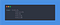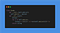# Motivation

Question1: Which Data Structure would you use to implement the navigation app from scratch?

Question2: We have the following flight diagram. The numbers express the flight prices in hundred dollars. Could you say the cheapest way to go from Berlin to Minsk?

The price is 15 (1500 dollars). In this case, we can calculate it with our hands after some trials, but what if we have a very huge amount of data? Then we need the help of the computer.

We can not implement such type of structure with Stack, Queue, Tree, etc. To express such type structures we use the Graph Data Structure.

# What is a Graph? Graph Terminology

A graph is an abstract data structure that is defined as the set of vertices and edges. It represents the adjacency and association between the vertices. Vertices are nodes and the edges show the connection between the Vertices (nodes).

Graph G can be shown as G = (V, E) mathematically. V is the set of the vertices:

And E is the set of the pair of vertices connected.

|V| is the number of the vertices, and |E| is the number of edges. What is the mathematical expression of the following graph?

Here we have 10 vertices, therefore, |V| = 10.

And, if G = (V,E)

V = {A,B,C,D,E,F,G,I,J,K}

E = {(A C),(A B),(B D),(C K), (KE),(E J),(J F),(F I),(I G),(G D)
,(K I),( AB),(K A),(J I),(J K),(FG),(I D),(A D)} |E| = 18

There are 2 main types of graphs: Directed and Undirected Graphs.

Undirected Graphs: the order of the vertices in the pairs in the Edge set does not make any sense. For example, in the graph example is given above, (G, F), and (F, G) pairs are the same. Undirected graphs generally are drawn with straight lines between the vertices.

Directed Graphs: the order of the vertices in the pairs in the Edge set to make sense and order is important. The following graph is directed.

Standard Assumption: A vertex is never adjacent to itself.

A maximum number of the edges of the undirected graph (order is not important):

A maximum number of the edges of a directed graph (order is important) is:

Degree of vertex: The degree of the graph shows the number of adjacent vertices

The degree of the vertex for the Undirected graph simply shows the number of adjacent vertices.

The degree of the vertex for the Directed graph is not one number. There are 2 numbers, the first shows the in (enters), the second number shows the out (exits). in/out

The path is an ordered sequence of vertices.

The Simple path of graph: Path with no repetition. For this graph, 1–2–5 can be an example of a simple path.

The cycle is the simple path, but the last vertex is the same as the first vertex. For example, 1–2–5–1

Both, Directed and Undirected graphs can be both weighted and unweighted. Weights are the values that represent the strength of the connection and are labeled on the edges. This could represent a distance, time, energy consumption associated with going from one vertex to the other.

Here is an example of the Cyclic Graph. It contains a cycle in the path. We can go from B to Athen D then again B. with the B-D-C-B cycle.

Here is the example of the Acyclic Graph. It does not contain any cycle.

# Graph Representation.

One can propose generally two ways of graph representation. The first method is called the Adjacency Matrix representation, the second way is the Adjacency List representation.

Adjacency Matrix shows the existence of the edges between two vertices. We choose the first vertex from the vertical line, and the second vertex from the horizontal line,

It’s the square matrix, if there’s a connection between two vertices, we assign the value 1, else 0

Be careful for the, directed graph, because (A, B) is not equal to (B, A), therefore, (A, B) will be 1, and (B, A) will be 0, because there is no connection.

Here is the example of the adjacency list, the first vertical set of blocks show our vertices, and each adjacency vertex connected to its neighbor as the technique of the linked list.

# Representation of the Graph in Python

For this article, we will work with the adjacency list. The Logic is quite simple, use the dictionary to represent the Graph. Each vertex is the key of the dictionary and each key has the value which is the list of the adjacency list. For example, the following dictionary represents the graph that you see just above.You can see the vertex 1 has the neighbors : [2,5], 1 is the key, and [2,5] is the value

But how to implement it? The following code block demonstrates the implementation of the Graph Data Structure. Let’s create the Graph class with the constructor which accepts the dictionary as an argument__str__ method helps us to print neatly our class object (toString() in java)

Let’s run our code and see the result

1. addVertex(self,new_vertex) which adds the new_vertex to the graph. For the first time, it will just add the new_vertex without its neighbors, then, if desired, the connections could be added using the add edge method which will be implemented. Method: We know that an adjacency list is a dictionary, so we must add the key to the dictionary called new_vertex with an empty list.

2. addEdge(self,pair_tuple) where pair_tuple = (v1,v2), which creates an edge and makes a connection between v1 and v2. Method: Find v1 from the keys of the dictionary and append v2 into the list of the key. Be careful!: If we are implementing the undirected graph, we must do the same thing for also the v2. Let’s make a connection between 6 and 1. Then the list of 6 must be only  and the list of 1 must be [2,5,6]

3. removeVertex(self,vertex) removes the vertex from the graph .Method: vertex from the dictionary and remove it using pop(vertex) function. We must also remove it from all the lists, it means all the connections must be removed. Let’s remove the vertex number 5 from all the graphs.